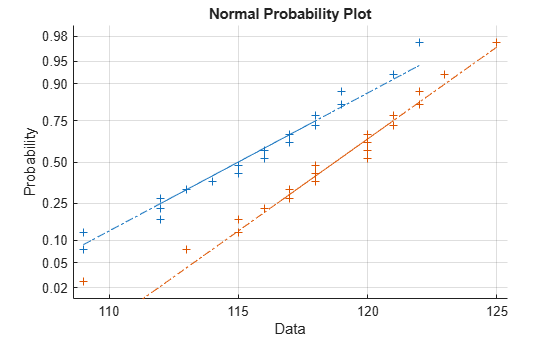Documentación

Esta página aún no se ha traducido para esta versión. Puede ver la versión más reciente de esta página en inglés.

## Hypothesis Testing

Hypothesis testing is a common method of drawing inferences about a population based on statistical evidence from a sample.

As an example, suppose someone says that at a certain time in the state of Massachusetts the average price of a gallon of regular unleaded gas was \$1.15. How could you determine the truth of the statement? You could try to find prices at every gas station in the state at the time. That approach would be definitive, but it could be time-consuming, costly, or even impossible.

A simpler approach would be to find prices at a small number of randomly selected gas stations around the state, and then compute the sample average.

Sample averages differ from one another due to chance variability in the selection process. Suppose your sample average comes out to be \$1.18. Is the \$0.03 difference an artifact of random sampling or significant evidence that the average price of a gallon of gas was in fact greater than \$1.15? Hypothesis testing is a statistical method for making such decisions.

This example shows how to use hypothesis testing to analyze gas prices measured across the state of Massachusetts during two separate months.

This example uses the gas price data in the file `gas.mat`. The file contains two random samples of prices for a gallon of gas around the state of Massachusetts in 1993. The first sample, `price1`, contains 20 random observations around the state on a single day in January. The second sample, `price2`, contains 20 random observations around the state one month later.

```load gas prices = [price1 price2];```

As a first step, you might want to test the assumption that the samples come from normal distributions. A normal probability plot gives a quick idea.

`normplot(prices)`Both scatters approximately follow straight lines through the first and third quartiles of the samples, indicating approximate normal distributions. The February sample (the right-hand line) shows a slight departure from normality in the lower tail. A shift in the mean from January to February is evident. A hypothesis test is used to quantify the test of normality. Since each sample is relatively small, a Lilliefors test is recommended.

`lillietest(price1)`
```ans = 0 ```
`lillietest(price2)`
```ans = 0 ```

The default significance level of `lillietest` is 5%. The logical 0 returned by each test indicates a failure to reject the null hypothesis that the samples are normally distributed. This failure may reflect normality in the population or it may reflect a lack of strong evidence against the null hypothesis due to the small sample size.

Now compute the sample means.

`sample_means = mean(prices)`
```sample_means = 1×2 115.1500 118.5000 ```

You might want to test the null hypothesis that the mean price across the state on the day of the January sample was \$1.15. If you know that the standard deviation in prices across the state has historically, and consistently, been \$0.04, then a z-test is appropriate.

`[h,pvalue,ci] = ztest(price1/100,1.15,0.04)`
```h = 0 ```
```pvalue = 0.8668 ```
```ci = 2×1 1.1340 1.1690 ```

The logical output `h` = 0 indicates a failure to reject the null hypothesis at the default significance level of 5%. This is a consequence of the high probability under the null hypothesis, indicated by the p value, of observing a value as extreme or more extreme of the z-statistic computed from the sample. The 95% confidence interval on the mean [1.1340 1.1690] includes the hypothesized population mean of \$1.15.

Does the later sample offer stronger evidence for rejecting a null hypothesis of a state-wide average price of \$1.15 in February? The shift shown in the probability plot and the difference in the computed sample means suggest this. The shift might indicate a significant fluctuation in the market, raising questions about the validity of using the historical standard deviation. If a known standard deviation cannot be assumed, a t-test is more appropriate.

`[h,pvalue,ci] = ttest(price2/100,1.15)`
```h = 1 ```
```pvalue = 4.9517e-04 ```
```ci = 2×1 1.1675 1.2025 ```

The logical output `h` = 1 indicates a rejection of the null hypothesis at the default significance level of 5%. In this case, the 95% confidence interval on the mean does not include the hypothesized population mean of \$1.15.

You might want to investigate the shift in prices a little more closely. The function `ttest2` tests if two independent samples come from normal distributions with equal but unknown standard deviations and the same mean, against the alternative that the means are unequal.

`[h,sig,ci] = ttest2(price1,price2)`
```h = 1 ```
```sig = 0.0083 ```
```ci = 2×1 -5.7845 -0.9155 ```

The null hypothesis is rejected at the default 5% significance level, and the confidence interval on the difference of means does not include the hypothesized value of 0. A notched box plot is another way to visualize the shift.

```boxplot(prices,1) h = gca; h.XTick = [1 2]; h.XTickLabel = {'January','February'}; xlabel('Month') ylabel('Prices (\$0.01)')```The plot displays the distribution of the samples around their medians. The heights of the notches in each box are computed so that the side-by-side boxes have nonoverlapping notches when their medians are different at a default 5% significance level. The computation is based on an assumption of normality in the data, but the comparison is reasonably robust for other distributions. The side-by-side plots provide a kind of visual hypothesis test, comparing medians rather than means. The plot above appears to barely reject the null hypothesis of equal medians.

The nonparametric Wilcoxon rank sum test, implemented by the function `ranksum`, can be used to quantify the test of equal medians. It tests if two independent samples come from identical continuous (not necessarily normal) distributions with equal medians, against the alternative that they do not have equal medians.

`[p,h] = ranksum(price1,price2)`
```p = 0.0095 ```
```h = logical 1 ```

The test rejects the null hypothesis of equal medians at the default 5% significance level.